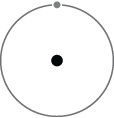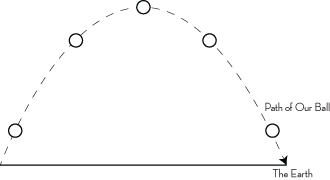Back Home

# Relativity & The Flat Earth

### Newton’s Laws of Motion

1. Every object in a state of uniform motion tends to remain in that state of motion unless an external force is applied to it.
2. The acceleration of an object as produced by a net force is directly proportional to the magnitude of the net force, in the same direction as the net force, and inversely proportional to the mass of the object. F=ma
3. For every action there is an equal and opposite reaction.

### Einstein’s Equivalence Principle

Einstein was fond of a form of inquiry known as thought experiments. In a thought experiment, a premise is assumed to deduct from it the possible consequences. One of these thoughts experiments in particular involved a solitary passenger on an elevator that is alone in the universe.

When the elevator starts accelerating upwards, a pull downwards is felt by the passenger. This is due to the passengers inertia. Recall that a body at rest tends to stay at rest. Einstein hypothesised that since this pull, caused by the momentum of the passenger fighting against his acceleration, is indistinguishable from gravitational influences, it is only logical to assume that they are one and the same phenomenon. Comparatively, another pseudo-force like this arises from a rotating reference frame, say if one is on a spinning carnival ride one would presumably feel a pull away from the center.

Consider the scenario of this elevator accelerating upwards at 9.81 m/s/s . If the passenger were to jump in the elevator while it was accelerating upwards at 9.81 m/s/s (the rate at which we are pulled to the earth by gravitational forces) he would observe the same effect as if he was jumping on the earth. An initial resistance would be felt; the ground, or elevator floor, would recede away from him at 9.81m/s/s until he has traveled enough distance to expend the force given by jumping. Then the elevator floor would rise up to meet him at 9.81m/s/s . This can be seen as an observational illusion: the elevator floor can be seen to either be accelerating upwards at 9.81m/s/s or the passenger could be seen as falling towards the floor. To the passenger - its indistinguishable! Einstein’s inductive leap here was the realization that perhaps the forces are indistinguishable because they are. They both are accelerating frames of reference. The idea that gravitation is a pseudo-force arising from a naive view (which is to say taking a non-inertial frame of reference as an inertial one or vice versa) is known as the equivalence principle.

In short, gravitational pull then is shown as an observational illusion hinging on our naive view of our frame of reference being inertial. Gravity is actually revealed as an inertial force (also known as a fictitious force).

### A Flat Earth Theory

First let us construct a Wish List, so that we can be sure to be fair when we claim we’ve shown sufficient proof for our model. A simple list of things we would like to say are true if we have indeed shown a model that says the earth is flat.

### Our Flat Earth Theory Wishlist:

• We would like it to be coherent with our previous interpretation or show it as an approximation
• Results from a shift in our shift in the observational language, which is to say we are just “looking at it wrong” and this can be disillusioned, much like the Einstein’s Equivalence principle or Galileo’s inertia.
• Show the Earth is Flat
• Based on and coherent with known laws and facts

### What do we mean when we say the Earth is flat?

Let us remove an obvious point of confusion and simply state that we talk about flat in a broad manner - we are not discussing mountains or valleys here. In similar fashion, we are not making the ludicrous claim that the Earth is two dimensional. What we do say is that it can be transversed via a straight line through space. We would also like to say that all such traversals are straight or can be seen as straight. It can be said to satisfy this if it can be shown to be parallel to another straight line in space along all such traversals.

### The Ferrari Effect

Let us build first from the base of Newton.Consider a theoretical object in a perfectly stable orbit around a theoretical planet in a traditional round earth manner. Remember from Newtons laws of motion: an object in motion tends to stay in motion and in the direction it is in motion. We can certainly say that the object in orbit that it feels no experimentally verifiable difference in force or pseudo-force - which is equivalent to saying it is experimentally not accelerating (and thus not changing direction or speed.) Remember, Einstein disillusioned our naive view of space based on the equivalence principle.

Our sight would lead us to believe this might be foolish, but if space is curved (and Relativity relies on the assumption that it is) it would be silly to not question our visual representation of space since by all accounts it appears as if our observational (and theoretical) language is ill equipped to deal with description of it.

We should assume that it is indeed travelling in a straight line as its experimental evidence points us to. The issue is with our naive view of geometry and space. Likewise we take the view that it is indeed in motion and not still.

Let’s interpret the ramifications of the statement: an object in orbit travels in a straight (and thus flat), line through space through further thought experiment. First, we can define our field of interest in that taking all such theoretical orbits of our planet and realize them rightly as flat, thus defining the bounding space of interest also to be flat. It follows, given any orbit of this planet to be flat, the planet itself is flat since it satisfies our definition of flatness.

Let us again venture into thought experiment: eject some pods towards the earth from one such of our imaginary satellites at regular intervals along our orbit such that they are in free fall. Again, we can assume these are straight lines extending below to a translatable location on the surface of the earth, its geolocation. We can say these lines are normal to the trajectory of the satellite and they are normal to the ground, thus making the lines parallel. Since the orbit is straight, and the orbit relates directly to the geographical locations it is above, we have come a long way to show the planet is also flat.Now let us consider what acceleration means. Acceleration by its nature means either a change in speed or direction, which is to say a change in velocity. So when we look at the parabola formed by a ball in motion we can recognize that it is for the most part accelerating - it changes both direction and speed. Now, let us examine the path if we remove the influence of gravity from our model as well as unbound the start and end points to allow it to move freely.

If gravity was not forcing the object downwards, it would then be travelling a straight path, parallel perhaps to our imaginary satellite and in this case tangent to the apex of our balls climb.

We can see by comparison between a theoretical object in orbit and our ball at the apex of its climb that if not affected by gravity it would travel a straight line. By repeating this experiment again and again with lower apexes of our ball, various orientations, and so on we see the earth itself, not just the paths of satellites, is flat.The effect of viewing the earth and it appearing round is known as the Ferrari Effect, based off of former Canadian Flat Earth Society President Leo Ferrari who first predicted this. This describes that effect.

Any honest judge will begrudgingly have to admit that I have shown that the flat earth theory directly follows from our laws of motion and coherence with relativity. Even worse is the realization that we would have been lead to relativity sooner if not for our strict faith-like belief in a round earth.

J Davis, American Flat Earth Society President